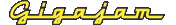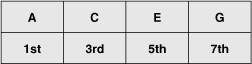EMT Lesson Three  How to create and play chords

# Formula for deriving chords from the major scale

It is common practise to describe the construction of a chord by naming each note in the chord by its number in the major scale.

Take the chord of Am7 (Aminor7) for instance. Its construction would be described as: 1, b3, 5, b7.

As you can see, when compared to the notes of the A major scale, the third note and the seventh notes are flattened.

Comparing this formula to the scale of A major:

The 1 in the scale is A.
The 3 in the scale is C# which is then flattened to C (b3).
The 5 is E.
The 7th note is G#, which is flattened to G (b7) as it is a minor chord.

The notes in the chord of Am7 are therefore:

A, C, E, G.

Figure 13. Chord of Aminor7 (Am7)   (Enlarge)